# NCERT Solutions for Class 11 Chemistry Some Basic Concepts of Chemistry Part 1## myCBSEguide App

Complete Guide for CBSE Students

NCERT Solutions, NCERT Exemplars, Revison Notes, Free Videos, CBSE Papers, MCQ Tests & more.

NCERT solutions for Class 11 Chemistry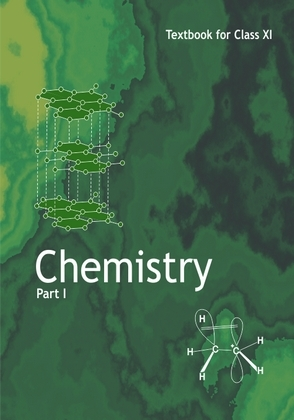## NCERT Class 11 Chemistry Chapter wise Solutions

• Chapter 1 – Some Basic Concepts of Chemistry
• Chapter 2 – Structure of Atom
• Chapter 3 – Classification of Elements and Periodicity in Properties
• Chapter 4 – Chemical Bonding and Molecular Structure
• Chapter 5 – States of Matter
• Chapter 6 – Thermodynamics
• Chapter 7 – Equilibrium
• Chapter 8 – Redox Reactions
• Chapter 9 – Hydrogen
• Chapter 10 – The s-Block Elements
• Chapter 11 – The p-Block Elements
• Chapter 12 – Organic Chemistry – Some Basic Principles and Techniques
• Chapter 13 – Hydrocarbons
• Chapter 14 – Environmental Chemistry

## NCERT Solutions for Class 11 Chemistry Some Basic Concepts of Chemistry Part 1

Chapter – Class 11 Chemistry Some Basic Concepts of Chemistry Part 1
1. Calculate the molecular mass of the following:

(i)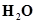(ii)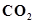(iii)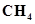2. Calculate the mass percent of different elements present in sodium sulphate (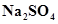).

3. Determine the empirical formula of an oxide of iron which has 69.9% iron and 30.1% dioxygen by mass.

4. Calculate the amount of carbon dioxide that could be produced when

(i) 1 mole of carbon is burnt in air.

(ii) 1 mole of carbon is burnt in 16 g of dioxygen.

(iii) 2 moles of carbon are burnt in 16 g of dioxygen.

5. Calculate the mass of sodium acetaterequired to make 500 mL of 0.375 molar aqueous solution. Molar mass of sodium acetate is 82.0245 g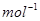6. Calculate the concentration of nitric acid in moles per litre in a sample which has a density, 1.41 g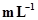and the mass per cent of nitric acid in it being 69%.

7. How much copper can be obtained from 100 g of copper sulphate (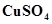)?

8. Determine the molecular formula of an oxide of iron in which the mass per cent of iron and oxygen are 69.9 and 30.1 respectively. Given that the molar mass of the oxide is 159.69 g.

9. Calculate the atomic mass (average) of chlorine using the following data: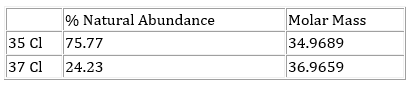10. In three moles of ethane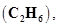calculate the following:

(i) Number of moles of carbon atoms.

(ii) Number of moles of hydrogen atoms.

(iii) Number of molecules of ethane.

11. What is the concentration of sugar (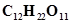) in mol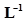if its 20 g are dissolved in enough water to make a final volume up to 2 L?

12. If the density of methanol is 0.793 kg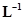, what is its volume needed for making 2.5 L of its 0.25 M solution?

13. Pressure is determined as force per unit area of the surface. The SI unit of pressure, Pascal is as shown below: 1Pa =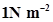If mass of air at sea level is 1034 g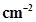, calculate the pressure in Pascal.

14. What is the SI unit of mass? How is it defined?

15. Match the following prefixes with their multiples: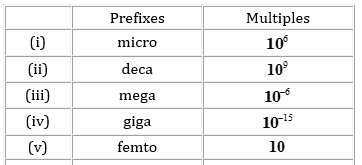16. What do you mean by significant figures?

17. A sample of drinking water was found to be severely contaminated with chloroform,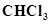, supposed to be carcinogenic in nature. The level of contamination was 15 ppm (by mass).

(i) Express this in percent by mass.

(ii) Determine the molality of chloroform in the water sample.

18. Express the following in the scientific notation:

(i) 0.0048

(ii) 234,000

(iii) 8008

(iv) 500.0

(v) 6.0012

19. How many significant figures are present in the following?

(i) 0.0025

(ii) 208

(iii) 5005

(iv) 126,000

(v) 500.0

(vi) 2.0034

## NCERT Solutions for Class 11 Chemistry Chapter 1 Some Basic Concepts of Chemistry

NCERT Solutions Class 11 Chemistry Some Basic Concepts of Chemistry Part 1 PDF (Download) Free from myCBSEguide app and myCBSEguide website. Ncert solution class 11 Chemistry includes text book solutions from both part 1 and part 2. NCERT Solutions for CBSE Class 11 Chemistry have total 14 chapters. 11 Chemistry NCERT Solutions in PDF for free Download on our website. Ncert Chemistry class 11 solutions PDF and Chemistry ncert class 11 PDF solutions with latest modifications and as per the latest CBSE syllabus are only available in myCBSEguide

## NCERT Solutions for Science Class 3rd to 12th

To download NCERT Solutions for class 11 Chemistry, Physics, Biology, History, Political Science, Economics, Geography, Computer Science, Home Science, Accountancy, Business Studies and Home Science; do check myCBSEguide app or website. myCBSEguide provides sample papers with solution, test papers for chapter-wise practice, NCERT solutions, NCERT Exemplar solutions, quick revision notes for ready reference, CBSE guess papers and CBSE important question papers. Sample Paper all are made available through the best app for CBSE students and myCBSEguide.com website.## Test Generator

Create Papers with your Name & Logo